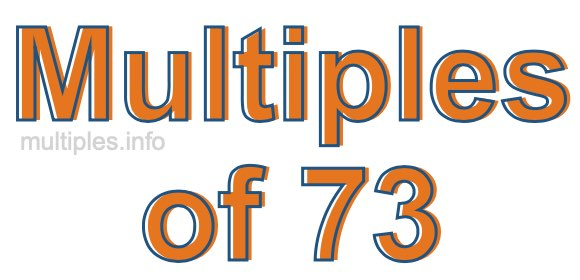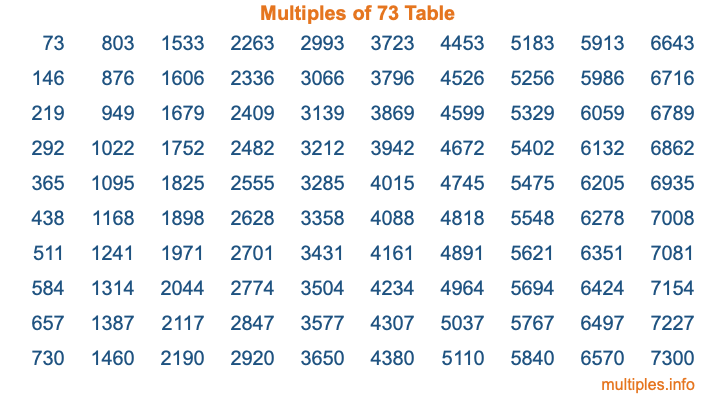Multiples of 73Welcome to the Multiples of 73 page. Here we will first teach you everything you will ever need to know about the multiples of 73, and then give you a study guide summary of everything we taught you to make sure you remember it all. Use this page to look up facts and learn information about the multiples of 73. This page will make you a multiples of seventy-three expert!

Definition of Multiples of 73
Multiples of 73 are all the numbers that when divided by 73 equal an integer. Each of the multiples of 73 are called a multiple. A multiple of 73 is created by multiplying 73 by an integer.

Therefore, to create a list of multiples of 73, you start with 1 multiplied by 73, then 2 multiplied by 73, then 3 multiplied by 73, and so on for as long as you want. Thus, the list of the first five multiples of 73 is 73, 146, 219, 292, and 365. To see a larger list of multiples of 73, see the printable image of Multiples of 73 further down on this page. We also have a category where you can choose any nth multiple of 73.

Multiples of 73 Checker
The Multiples of 73 Checker below checks to see if any number of your choice is a multiple of 73. In other words, it checks to see if there is any number (integer) that when multiplied by 73 will equal your number. To do that, we divide your number by 73. If the the quotient is an integer, then your number is a multiple of 73.

Is  a multiple of 73?

Least Common Multiple of 73 and ...
A Least Common Multiple (LCM) is the lowest multiple that two or more numbers have in common. This is also called the smallest common multiple or lowest common multiple and is useful to know when you are adding our subtracting fractions. Enter one or more numbers below (73 is already entered) to find the LCM.

Check out our LCM Calculator if you need more details about the Least Common Multiple or if you need the LCM for different numbers for adding and subtraction fractions.

nth Multiple of 73
As we stated above, 73 is the first multiple of 73, 146 is the second multiple of 73, 219 is the third multiple of 73, and so on. Enter a number below to find the nth multiple of 73.

th multiple of 73

Multiples of 73 vs Factors of 73
73 is a multiple of 73 and a factor of 73, but that is where the similarities end. All postive multiples of 73 are 73 or greater than 73. All positive factors of 73 are 73 or less than 73.

Below is the beginning list of multiples of 73 and the factors of 73 so you can compare:

Multiples of 73: 73, 146, 219, 292, 365, etc.

Factors of 73: 1, 73

As you can see, the multiples of 73 are all the numbers that you can divide by 73 to get a whole number. The factors of 73, on the other hand, are all the whole numbers that you can multiply by another whole number to get 73.

It's also interesting to note that if a number (x) is a factor of 73, then 73 will also be a multiple of that number (x).

Multiples of 73 vs Divisors of 73
The divisors of 73 are all the integers that 73 can be divided by evenly. Below is a list of the divisors of 73.

Divisors of 73: 1, 73

The interesting thing to note here is that if you take any multiple of 73 and divide it by a divisor of 73, you will see that the quotient is an integer.

Multiples of 73 Table
Below is an image of the first 100 multiples of 73 in a table. The table is in chronological order, column by column. The first column has the first ten multiples of 73, the second column has the next ten multiples of 73, and so on.The Multiples of 73 Table is also referred to as the 73 Times Table or Times Table of 73. You are welcome to print out our table for your studies.

Negative Multiples of 73
Although not often discussed or needed in math, it is worth mentioning that you can make a list of negative multiples of 73 by multiplying 73 by -1, then by -2, then by -3, and so on, to get the following list of negative multiples of 73:

-73, -146, -219, -292, -365, etc.

Multiples of 73 Summary
Below is a summary of important Multiples of 73 facts that we have discussed on this page. To retain the knowledge on this page, we recommend that you read through the summary and explain to yourself or a study partner why they hold true.

There are an infinite number of multiples of 73.

A multiple of 73 divided by 73 will equal a whole number.

73 divided by a factor of 73 equals a divisor of 73.

The nth multiple of 73 is n times 73.

The largest factor of 73 is equal to the first positive multiple of 73.

73 is a multiple of every factor of 73.

73 is a multiple of 73.

A multiple of 73 divided by a divisor of 73 equals an integer.

73 divided by a divisor of 73 equals a factor of 73.

Any integer times 73 will equal a multiple of 73.

Multiples of a Number
Here you can get the multiples of another number, all with the same attention to detail as we did for multiples of 73 on this page.

Multiples of
Multiples of 74
Did you find our page about multiples of seventy-three educational? Do you want more knowledge? Check out the multiples of the next number on our list!